# NI PXIe-4844 Optical Sensor Interrogator Scaling Formulas for FBG Sensors

Publish Date: Jan 22, 2016 | 1 Ratings | 5.00 out of 5 | Print | Submit your review

## Overview

Optical sensing can benefit many applications, whether the application needs to span long distances, immunity to EMI and high voltages or the ability to take multiple measurements on a single cable. The PXIe-4844 optical sensor interrogator enables you to take advantage of these benefits as the best in class static interrogator. However, the interrogator is only one piece of an optical sensing system. Another important element in an optical sensing system is the FBG sensors. In order to ensure a properly setup optical sensing system, scaling formulas are required to be input to translate the shift in FBG to a strain or temperature value. This whitepaper will give you the appropriate scaling formulas to be entered into NI-OSI Explorer to ensure you receive the highest quality, accurate and reliable measurements.

### 1. FBG Sensors

Choose from a wide variety of FBG optical sensor, ranging from standard off-the-shelf strain gages to custom downhole oil and gas pressure transducers. You can daisy chain sensors including strain and temperature in any order by using optical connectors or splicing bare fibers with a fusion splicer. You can obtain sensors individually or in a preassembled array to meet your exact requirements.

The method for installing and using FBG optical sensors is very similar to the established methods used for electrical sensors. You can glue, weld, and embed FBG sensors while having fewer cables to manage with no noise, isolation, and/or shielding considerations to address. A variety of cabling exists, from simple low-cost to rugged deep sea cables, meeting the most extreme and rigid requirements.

### 2. Setup

Below are the scaling formulas that can be directly copied into NI-OSI Explorer once the appropriate variables are entered in (if applicable). Before getting to this step, let’s ensure a few things are completed first.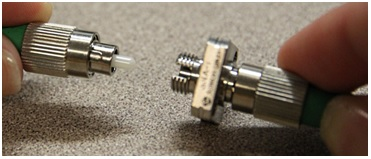1. Connect the sensor to the FC/APC Bulkead Adapter.

2. Connect the FC/APC to LC/APC Adapter Cable to the FC/APC Bulkhead Adapter.

3. Plug the FC/APC to LC/APC Adapter Cable into the PXIe-4844 Optical Sensor Interrogator.4. Connect more sensors together with the FC/APC Bulkhead Adapter or repeat steps 1-3.

5. Open NI-OSI Explorer, the sensor(s) will be displayed, match the measured wavelength with the nominal wavelength from the vendor specification sheet.

6. Click on a sensor and choose the appropriate sensor measurement.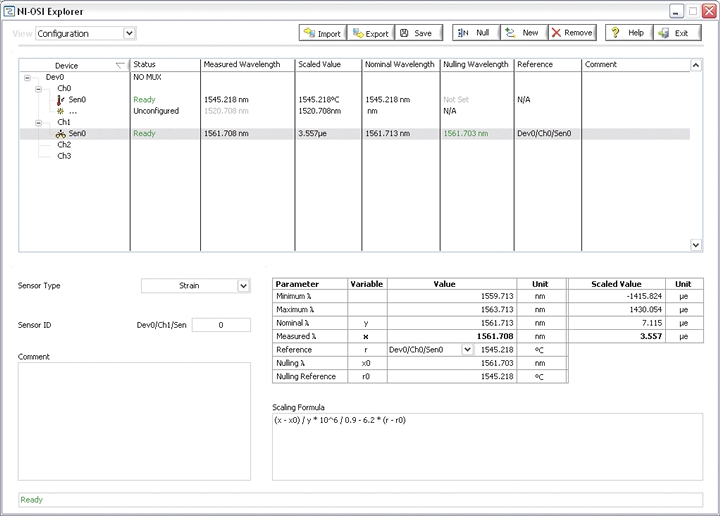### 3. Scaling Formulas

Each sensor below includes the vendor scaling formula, the NI-OSI scaling formula, and any clarifying variables.

For all strain FBGs, the scaling formula includes temperature compensation. In order to perform temperature compensation a second FBG (temperature) will need to be used. The Temperature FBG will be a reference to the strain FBG in NI-OSI Explorer.

Common terms used in all sensors are below. These terms can be entered in as shown.

• x – Measured Wavelength. This is the current wavelength of the FBG.
• y – Nominal Wavelength. This is the wavelength determined by the sensor vendor.
• x0 – Nulling Wavelength. This is the wavelength when the sensor was nulled.
• r – Reference Sensor Value. This is the current value of the temperature FBG.
• r0 – Nulling Reference Sensor Value. This is the value of the temperature FBG when the sensor was nulled.

Common variables used in sensors are below. These variables need to have a number entered in.

• Gage Factor – The value will be in the vendor specification sheet. Enter in this number for gage factor.
• Gage Constant 1 - The value will be in the vendor specification sheet. Enter in this number for gage constant 1.
• CTE of Test Specimen – This value is user defined and refers to the material the FBG is adhered to.
• Gage Constant 2 - The value will be in the vendor specification sheet. Enter in this number for gage constant 2.
• Nominal Wavelength Temp – The value will be in the vendor specification sheet. Enter in this number for nominal wavelength temp.
• Temperature Sensitivity – The value will be in the vendor specification sheet. Enter in this number for the temp sensitivity.
• CTE of Temperature Probe – This value will be in the vendor specification sheet. Enter in this number for the CTE of temperature probe.
• Calibration Coefficient 3 – This value will be in the vendor specification sheet. Enter in this number for calibration coefficient 3.
• Calibration Coefficient 2 - This value will be in the vendor specification sheet. Enter in this number for calibration coefficient 2.
• Calibration Coefficient 1 - This value will be in the vendor specification sheet. Enter in this number for calibration coefficient 1.
• Calibration Coefficient 0 - This value will be in the vendor specification sheet. Enter in this number for calibration coefficient 0.
• Wavelength Offset - This value will be in the vendor specification sheet. Enter in this number for the wavelength offset.
• Offset - This value will be in the vendor specification sheet. Enter in this number for the offset.

### Strain

 Sensor Use Case Scaling Formula os3110 FBG Optical Strain Gage, Spot Weld NI-OSI ε=((x-x0)/(y))*((1*10^6)/(Gage Factor))-((r-r0)*(Gage Constant 1/Gage Factor)+CTE of Test Specimen-Gage Constant 2) NI-OSI Ex. ((x-x0)/(y))*((1*10^6)/(0.89))-((r-r0)*(6.156/0.89)+13-0.70) Vendoros3120 FBG Optical Strain Gage, Epoxy Mount NI-OSI ε=((x-x0)/(y))*((1*10^6)/(Gage Factor))-((r-r0)*(Gage Constant 1/Gage Factor)+CTE of Test Specimen-Gage Constant 2) NI-OSI Ex. ((x-x0)/(y))*((1*10^6)/(0.89))-((r-r0)*(6.156/0.89)+13-0.70) Vendor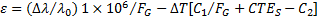os3150 Rugged FBG Optical Strain Gage NI-OSI ε=((x-x0)/(y))*((1*10^6)/(Gage Factor))-((r-r0)*(Gage Constant 1/Gage Factor)+CTE of Test Specimen-Gage Constant 2) NI-OSI Ex. ((x-x0)/(y))*((1*10^6)/(0.811))-((r-r0)*(6.156/0.811+13-0.70) Vendoros3155 Rugged FBG Optical Strain Gage with Integrated Temperature Compensation NI-OSI (Strain) ε=(1*10^6)*((((x-x0)/(y))-((r-r0)/(Nominal Wavelength Temp)))/(Gage Factor))-(((r-r0)/(Nominal Wavelength Temp))/(Temperature Sensitivity))*(CTE of Test Specimen-CTE of Temperature Probe) NI-OSI (Temp) T=(x-x0)+(Temperature of Environment) NI-OSI Ex. (S) (1*10^6)*((((x-x0)/(y))-((r-r0)/(1582.214)))/(0.807))-(((r-r0)/(1582.214))/(0.00001833))*(13-16) NI-OSI Ex. (T) (x-x0)+22 Vendor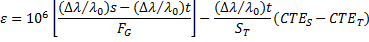os3200 Non-metallic FBG Optical Strain Gage NI-OSI ε=((x-x0)/(y))*((1*10^6)/(Gage Factor))-((r-r0)*(Gage Constant 1/Gage Factor)+CTE of Test Specimen-Gage Constant 2) NI-OSI Ex. ((x-x0)/(y))*((1*10^6)/(0.796))-((r-r0)*(6.156/0.796)+13-0.70) Vendor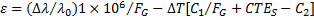os3600 Embeddable Long-gage Strain Sensor NI-OSI ε=(((x-x0)/(y))*((1*10^6)/(Gage Factor)) )-(((r-r0)/(Nominal Wavelength Temp))*((1*10^6)/(Gage Constant 1)) )+(CTE of Test Specimen-Gage Constant 2)*(1*10^3 )*((r-r0)/(Temperature Sensitivity)) NI-OSI Ex. (((x-x0)/(y))*((1*10^6)/(0.786)) )-(((r-r0)/(1551.756))*((1*10^6)/(0.796)) )+(13-10.1)*(1*10^3 )*((r-r0)/(23.8)) Vendor N/A os3610 Surface-mount Long-gage Strain Sensor NI-OSI ε=(((x-x0)/(y))*((1*10^6)/(Gage Factor)))-(((r-r0)/(Nominal Wavelength Temp))*((1*10^6)/(Gage Constant 1)) )+(CTE of Test Specimen-Gage Constant 2)*(1*10^3 )*((r-r0)/(Temperature Sensitivity)) NI-OSI Ex. (((x-x0)/(y))*((1*10^6)/(0.840)))-(((r-r0)/(1521.818))*((1*10^6)/(0.813)) )+(13-11)*(1*10^3 )*((r-r0)/(22.0)) Vendor N/A

### Temperature

 Sensor Use Case Scaling Formula os4100 Temperature Compensation Gage NI-OSI T=(1*10^3 )*((x-x0)/(Temperature Sensitivity) NI-OSI Ex. (1*10^3 )*((x-x0)/(28.9) Vendoros4210 Temperature Probe NI-OSI T=(Calibration Coefficient 3)*(((x-x0)+(Wavelength Offset) )^3 )+(Calibration Coefficient 2)*(((x-x0)+(Wavelength Offset) )^2 )+(Calibration Coefficient 1)*((x-x0)+(Wavelength Offset) )+(Calibration Coefficient 0) NI-OSI Ex. (3.7529852856878300)*(((x-x0)+(7.9227) )^3 )+(-17397.533932784500)*(((x-x0)+(7.9227) )^2 )+(26883059.46686950)*((x-x0)+(7.9227) )+(-13846814009.7684) Vendor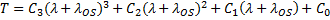os4230 Ruggedized Temperature Probe NI-OSI T=(Calibration Coefficient 3)*(((x-x0)+(Wavelength Offset) )^3 )+(Calibration Coefficient 2)*(((x-x0)+(Wavelength Offset) )^2 )+(Calibration Coefficient 1)*((x-x0)+(Wavelength Offset) )+(Calibration Coefficient 0) NI-OSI Ex. (3.7529852856878300)*(((x-x0)+(7.9227) )^3 )+(-17397.533932784500)*(((x-x0)+(7.9227) )^2 )+(26883059.46686950)*((x-x0)+(7.9227) )+(-13846814009.7684) Vendoros4280   Ruggedized Probe w/ Thermocouple Head NI-OSI T=(Calibration Coefficient 3)*(((x-x0)+(Wavelength Offset) )^3 )+(Calibration Coefficient 2)*(((x-x0)+(Wavelength Offset) )^2 )+(Calibration Coefficient 1)*((x-x0)+(Wavelength Offset) )+(Calibration Coefficient 0) NI-OSI Ex. (3.7529852856878300)*(((x-x0)+(7.9227) )^3 )+(-17397.533932784500)*(((x-x0)+(7.9227) )^2 )+(26883059.46686950)*((x-x0)+(7.9227) )+(-13846814009.7684) Vendoros4310 Temperature Sensor NI-OSI T=(Calibration Coefficient 3)*(((x-x0)+(Wavelength Offset) )^3 )+(Calibration Coefficient 2)*(((x-x0)+(Wavelength Offset) )^2 )+(Calibration Coefficient 1)*((x-x0)+(Wavelength Offset) )+(Calibration Coefficient 0) NI-OSI Ex. (3.4716563220263200)*(((x-x0)+(12.0233) )^3 )+(-16427.242229277400)*(((x-x0)+(12.0233) )^2 )+(25910320.73981810)*((x-x0)+(12.0233) )+(-13622634512.06860) Vendoros4330 Non-metallic Epoxy Mount Temperature Sensor NI-OSI T=(Calibration Coefficient 3)*(((x-x0)+(Wavelength Offset) )^3 )+(Calibration Coefficient 2)*(((x-x0)+(Wavelength Offset) )^2 )+(Calibration Coefficient 1)*((x-x0)+(Wavelength Offset) )+(Calibration Coefficient 0) NI-OSI Ex. (3.4716563220263200)*(((x-x0)+(12.0233) )^3 )+(-16427.242229277400)*(((x-x0)+(12.0233) )^2 )+(25910320.73981810)*((x-x0)+(12.0233) )+(-13622634512.06860) Vendoros4350 Armored Cable, Flange Mount Temperature Sensor NI-OSI T=(Calibration Coefficient 3)*(((x-x0)+(Wavelength Offset) )^3 )+(Calibration Coefficient 2)*(((x-x0)+(Wavelength Offset) )^2 )+(Calibration Coefficient 1)*((x-x0)+(Wavelength Offset) )+(Calibration Coefficient 0) NI-OSI Ex. (3.4716563220263200)*(((x-x0)+(12.0233) )^3 )+(-16427.242229277400)*(((x-x0)+(12.0233) )^2 )+(25910320.73981810)*((x-x0)+(12.0233) )+(-13622634512.06860) Vendoros4400   Temperature Sensing Cable (Each FBG will have a scaling formula) NI-OSI T=(Calibration Coefficient 3)*(((x-x0)+(Wavelength Offset) )^3 )+(Calibration Coefficient 2)*(((x-x0)+(Wavelength Offset) )^2 )+(Calibration Coefficient 1)*((x-x0)+(Wavelength Offset) )+(Calibration Coefficient 0) NI-OSI Ex. (3.4716563220263200)*(((x-x0)+(12.0233) )^3 )+(-16427.242229277400)*(((x-x0)+(12.0233) )^2 )+(25910320.73981810)*((x-x0)+(12.0233) )+(-13622634512.06860) Vendor### Displacement

 Sensor Use Case Scaling Formula os5100   Displacement Gage NI-OSI D=(Gage Factor)*((x-x0)-(r-r0) )+Offset NI-OSI Ex. (6.1573)*((x-x0)-(r-r0) )+(-15.72) Vendoros5500 Long Range Displacement Gage NI-OSI D=(Calibration Coefficient 3)*((x-x0)^3)+(Calibration Coefficient 2)*((x-x0)^2)+((Calibration Coefficient 1)*(x-x0)+(Calibration Coefficient 0) NI-OSI Ex. (-0.00145)*((x-x0)^3)+(0.0260)*((x-x0)^2)+((16.641)*(x-x0)+(-25.25) Vendor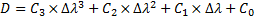### Ratings

Rate this document